# Modelling Approach for Fast Simulation and Optimization of Large District Heating Networks

Modelling Approach for Fast Simulation and Optimization of Large District Heating Networks

Vittorio VerdaElisa Guelpa

Politecnico di Torino, Corso Duca degli Abruzzi 24, Torino, Italy

Corresponding Author Email:
vittorio.verda@polito.it
Page:
397-403
|
DOI:
https://doi.org/10.18280/ti-ijes.632-441
26 January 2019
|
Accepted:
6 May 2019
|
Published:
30 June 2019
| Citation

OPEN ACCESS

Abstract:

District heating (DH) networks are becoming in the last year a crucial energy infrastructure, as they allow significant increase in the share of renewables either directly used or through conversions from a multi-energy system perspective. In this framework, network simulations are crucial for analyzing the effects of the modifications in DH systems and implement improvement or optimization strategies. Two peculiar aspects which refer to DH networks are related with the changes in fluid flow distribution and the thermal transients that occur during operation, which might have significant effects, especially in large systems. These occur for instance when the circulating mass flow rate changes due to peak demands, e.g. when the heating systems are switched on in the morning, when malfunctions occur or when the network is used as a thermal storage.

DH networks can be modelled through physical approaches, which considers fluid flow and transient heat transfer relying on numerical methods. In case of large networks or when super-real time simulations or optimizations are required, equivalent topology descriptions can be usefully applied to reduce the required computational resources. The goal of this work consists in proposing a modeling approach able to reduce the computational time required for simulating a network, which act on the fluid flow problem and the thermal problem. The approach is applied to the Turin district heating network, which is the largest network in Italy. Results show that the computational time is reduced of three orders of magnitude with small deviations in the results compared with the full model.

Keywords:

district heating, network models, reduced models, system operation

1. Introduction

District heating has significantly evolved in the last years. The main trends are related with the concepts of fourth generation DH  (and there are already ideas for the fifth generation ) and the multi-energy systems. 4th generation DH involves various technical innovations, all finalized to increasing system efficiency, reducing CO2 emissions and keeping costs affordable. These innovations are mainly related to the reduction in supply and return temperatures , increase in renewable  and waste heat penetration , increase the energy storage capacity and involve the end-users. Multi-energy systems , instead, are based on the idea of connecting the various energy networks (electric grid, gas network and district heating/cooling network) in order to convert, and somehow store, energy in different forms. In this perspective district heating can be used to convert excess electricity available from renewables into heat which can be delivered to the end-users or stored. This is known as the power-to-heat  and can be performed through heat pumps or electric boilers. District heating make it more convenient with respect to single houses.

Optimal management [8-9] is of extreme importance to achieve ambitious targets in terms of primary energy consumption, CO2 emissions and economic benefits from selling heat and electricity. In this framework, modelling of DH systems allows one to analyse the effects of operational strategies such as thermal demand-side management [10-11], virtual storage, power-to-heat, waste heat recovery, but also the installation of thermal storage units [12-13] of the integration of renewable sources.

All these strategies affect the thermal and fluid-dynamic behaviour of the system and their results depend on the network topology, especially in the case of large networks. This is mainly due to the thermal transients, which cause delays between the thermal demand and the thermal load. In other words, the total demand profile at the end-users is different than the total load profile at the plants. This means that the performance achieved might be significantly different depending on the position (and characteristics) of the energy sources and end-users.

Physical models rely on the definition and solution of the physical problem on the entire DH network pipeline. Physical models require the topological description of the network and allows evaluating mass flows and temperature distributions in the network. The non-linear problem that characterize the DHN fluid-dynamic is solved by numerical methods that are often computationally expensive.

In black box approaches, the nonlinearity is overcome using standard transfer functions or neural networks. In order to build and validate these kinds of models, a certain amount of experimental data, or simulation results, are necessary [14-15]. The main drawback of these methods is related with the fact that information on the topology is lost, therefore only a global evaluation of the system is provided.

When extended DH networks are considered, the computational cost becomes a crucial characteristic of a simulation tool. This is due to the large number of nodes and branches included in the network. Indeed the solution of the thermal fluid-dynamic problem may require very high computational resources. An alternative approach has to be used in these cases. Two approaches that can be combined together are presented in this paper: a reduction of the fluid dynamic problem that can be applied to loop networks and a reduced model of the energy equation. The first approach is particularly suitable for the simulation of large transport networks, where loops are frequently used. The second approach is suitable for the simulation of distribution networks and to take the effects of these networks into account at a larger scale.

2. Full Physical Model for DH Networks

Physical models of DH networks are based on a one-dimensional description of the network. Mathematical description of its topology is obtained using graph theory . Each pipe is considered as a branch bounded by two nodes and with a conventional verse. In this way the conventional inlet node and outlet node can be defined. The mass flow rate is then positive when it flows in the same verse as the conventional verse and negative when it flows in the opposite verse. The incidence matrix (A) with as many rows as the number of nodes and as many columns as the number of branches describes the connection between the various branches. A general element Aij is equal to 1 or -1 if the branch j enters or exits the node i and 0 otherwise.

The thermal fluid-dynamic model adopted in this work considers the mass and momentum equations in steady state and the energy conservation equation in transient conditions. The mass balance equation applied to all nodes is written as:

A⋅G+Gext=0 (1)

where, G is the vector containing the mass flow rates in the branches (to be determined) and Gext is the vector of the mass flow rates exchanged with the extern, i.e. the thermal plants and the buildings (or group of buildings, depending on the representation) when an open network is considered (only the supply network or the return network). The momentum equation is written for each branch:

G=Y⋅AT⋅P+Y⋅Δppumps (2)

Eq. (2) is written considering the hypothesis of incompressible fluid. The vector P contains the total pressures in the nodes (to be determined), Δppumps is the vector of pressure rises which terms are different than zero in the branches where active pumps are located, the matrix Y is a diagonal matrix; each term is the fluid dynamic conductance of the corresponding branch:

$Y=R^{-1}=[\frac{1}{2}\frac{G}{ρS^2}(\frac{f}{D}L+\sum_{k}β_k )]^{-1}$ (3)

where, S is the pipe cross section, f the friction factor, L the pipe length, D the pipe diameter, ρ the fluid density, and β a coefficient accounting for local pressure losses occurring in special components such as flow splitters, mixers, etc.

The fact that the terms in Y depend on the mass flow rates makes the equations non-linear. A proper algorithm for solving the equation set is thus necessary.

The energy equation is written for each node, as:

$M\dot{T}+KT=g$ (4)

where, T is the vector containing the temperatures in the nodes (to be determined), M and K are the mass matrix and the stiffness matrix, while g is the vector containing the known terms.

When considering DH operation, three different issues, which cause an increase in the computational time, might arise:

1) Equations (1) and (2) are coupled by means of the vector G. In the case of three-shape networks (without loops), the mass flow rates are obtained from direct solution of the mass conservation equations. Using this result, pressures in the nodes (if needed) are obtained from the momentum equation, which is a linear equation. Coupling becomes strong in the case the network presents loops. In this case, the momentum equation depends in a non-linear form from the mass flow rates, which are unknown. The two matrix equations must be solved together using a proper iterative algorithm. In the case of the solution method presented in this paper, the SIMPLE algorithm  is used.

2) Thermal transients might last various hours, especially in large networks. In these cases, simulations require multiple iterations of the energy equation.

3) When optimizations are performed or multiple scenarios are investigated, the issues 1) and 2) are further strengthened.

In this work, two different approaches aiming at solving the aforementioned issues are presented. These are two model reduction techniques aiming at considering the effects of network topology, but with reduced computational costs. An application to the Turin district heating network is proposed.

3. Compact Approach for DH Network Modelling

The approach presented in this paper is finalized to reduce the computational cost for modeling large district heating networks. As already discussed, information on the mass flow rates and temperature distributions is generally necessary to properly examine design or operational improvements. Reductions in computational costs should be therefore obtained both on the fluid dynamic problem and thermal problem. Figure 1 shows a schematic of the approach presented in this paper, which acts in parallel on the two problems.  The full approach is described in the following sections.

## 1.pngFigure 1. Schematic of the compact modelling approach

3.1 Compact fluid dynamic model

The fact that a network presents one or more loops causes the needs of an iterative algorithm for the solution. A possible approach for significantly reduce the computational time consists in transforming a loop network into a tree-shape network. This result can be achieved by using a black box model, which evaluates the water flow rate in a loop branch. That branch can be then eliminated and the corresponding mass flow rate assigned as a boundary condition in the inlet and outlet nodes. In particular, the same value as the mass flow rate should be assigned to the vector Gext in the row corresponding to the inlet node (either positive or negative), while the value with opposite sign should be assigned to the row corresponding to the outlet node. As an example, Figure 2a shows a network with 3 loops. The equivalent tree-shape network is obtained by eliminating a branch in each loop, as shown in Figure 2b, where the equivalent mass flow rates in the nodes are indicated.

In the present work, a linear model is considered to evaluate the mass flow rates in the loop branches. The flow circulation mainly depends on the thermal load and the way it is shared between the various thermal plants. For this reason, the mass flow rates exiting the various thermal plants are taken as the independent variables in the linear model. This procedure allows significant reduction in the computational resources necessary for solving the fluid dynamic problem, but it does not provide the pressure distribution along the network. Such result can be obtained by applying the momentum equation, which is solved as a linear equation set as the mass flow rates are fully known.

## 2.pngFigure 2. Procedure for the simplification of looped network

3.2 Compact thermal model

The computational time requested to solve the thermal model can be significantly reduced by grouping together the demand of all buildings served by a single distribution network, while the transport network is fully represented. In the application to the Turin district heating network, the approach presented below allows one reducing the number of nodes from about 60000 to about 2000, which means that the computational time is reduced of a factor 900.

A schematic of the modeling reduction approach is presented in figure 3. For each distribution network, the energy equation is applied to a limited number of branches. These are not directly associated with physical portions of the pipeline, but are “equivalent branches” that allow to obtain the same evolution of the thermal demands as the real network.

Each branch is characterized by a specific value of the mass term and the stiffness term appearing in equation 4. This means that the model has a number of parameters equal to twice the number of equivalent branches. Once the number of branches is selected, the various buildings are assigned to the equivalent branches using a clustering approach. A k-mean algorithm is used with the aim of determining the best clustering creation, once the number of cluster is defined. The mass and the stiffness terms of each cluster are determined on the basis of two parameters: 1) the equivalent distance of the building from the point connecting the distribution network to the transport network; 2) the equivalent pipe diameter.

## 3.png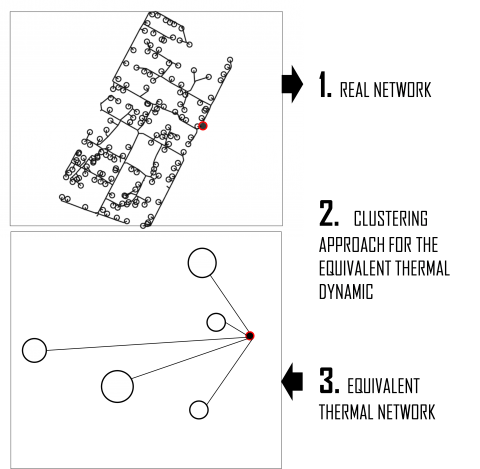Figure 3. Procedure for the thermal simplification of district heating networks

4. Case Study

## 4.png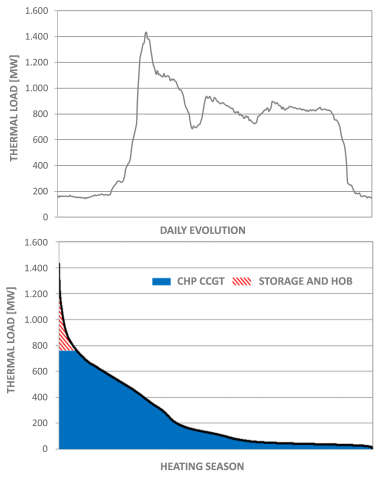Figure 4. Thermal load profile in a typical winter day and annual duration curve of the Turin DH

The Turin district heating serves over 600000 inhabitants and a total volume of buildings over 60 million m3, which make it the largest network in Italy and one of the largest in Europe. The maximum load is over 1.4 GW and the annual energy request is about 2160 GWh. This is mainly covered by three combined cycles operating in cogeneration mode, able to supply up to about 760 MW. The remaining load is covered by thermal various storage units with a total capacity of 15000 m3 and four heat-only plants with a total capacity of 1000 MW. Figure 4 shows the daily profile of the thermal load in a typical winter day and the annual duration curve. About 96 % of the energy is below the cogeneration capacity. The remaining portion is covered by storage units (which are charged using the cogeneration systems) and boilers. Despite these performances, it is desirable to reduce the thermal peak load and make the profile more flexible. This allows one to further increase the percentage of heat supplied through cogeneration and increase the ability of combined cycles to compete on the electricity market.

The pipeline has a total length of over 550 km. The working fluid is super-heated water, which is supply at the nominal temperature of 120 °C. The return temperature varies between 70 °C in typical winter days to about 40 °C in middle seasons, depending on the thermal demand. Load is adjusted by acting on the mass flow rate circulating the network. This is achieved through pumping stations located in the plants and booster pumps located in various points of the network , where pumps with variable speed are installed.

The district heating network is represented as composed of two parts: the transport network and the distribution networks. The transport network connects the thermal plants with the various areas of the town and is composed by the pipes with diameters larger than 200 mm. The transport network is about 70 km long and includes 9 loops. These are used to reduce the effects of possible failures allow a more effective management. A schematic of the transport network is shown in figure 5. The distribution networks connect the transport network with the various buildings located in each area. In the Turin network there are 182 distribution networks. The representation of the entire network requires more than 60000 nodes.

## 5.pngFigure 5. Schematic of the Turin transport network

5. Results

## 5.1 Compact fluid-dynamic model

In order to test the method, the two models (full model and compact model) are applied to the transport network of the Turin district heating. The 9 mass flow rates in the loop branches are determined using the linear model trained using the results of previous simulations performed by means of the full model and considering various different thermal load levels with different plant configurations. Figure 6 shows the mass flow rates in the loop branches in two operating conditions, corresponding to 30 % and 90 % of the design thermal load. Results show that the compact model is able to predict the mass flow rates with a maximum error always lower than 4 %. The impact on the mass flow rates circulating the network is quite limited, in fact the average deviations range from 0.1 % to 0.3 %, depending on the thermal load. The impact on load prediction is always lower than 1.5 %, which is a good result. In the specific application, the network is represented by means of 968 nodes. With this size, the computational time using the reduced model is three order of magnitude smaller than that required using the full model.

## 6.png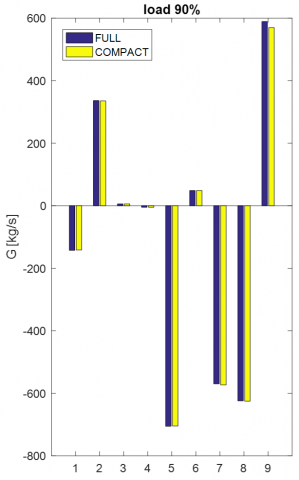Figure 6. Comparison of the mass flow rates in the loop branches obtained using the full model and the reduced model

## 5.2 Compact thermal model

A medium size distribution network is considered in this analysis. This is represented in figure 7.

The buildings connected to the network are represented as squares, which are grouped into 5 clusters. Colors in the figure identify the clusters. The equivalent network is shown in Figure 8.

## 7.png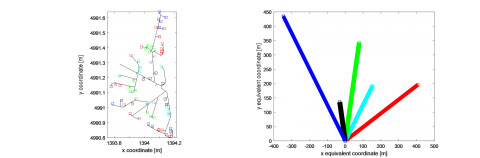Figure 7. Topology of a medium size distribution network and building distribution between 5 clusters

## 8.pngFigure 8. Equivalent network

## 9.pngFigure 9. Comparison of the load profile for the distribution network calculated using the thermal model applied to the full network and to the equivalent network

Figure 9 shows the evolutions of the thermal load for the entire distribution network calculated considering the entire pipeline and the equivalent network. The evolutions are similar, with deviations lower than 4%. In particular, the reduced model tends to overestimate the thermal losses in the network. Further improvements in this regard can be achieved by adopting a different clustering approach, which optimize the distribution of buildings within the clustering while minimizing the deviation between the profiles calculated with the full representation and with the reduced model. Various profiles corresponding with different external temperatures can be also considered in order to make the model more suitable for extrapolations.

6. Applications

Design and management solutions for district heating systems are often examined using black box models, without considering the effects of network topology. These effects might be not negligible. As an example, when heat pumps are installed in district heating systems for power-to-heat purposes, the overall performance depends on the selected location, due to the different temperature distribution.

## 10.png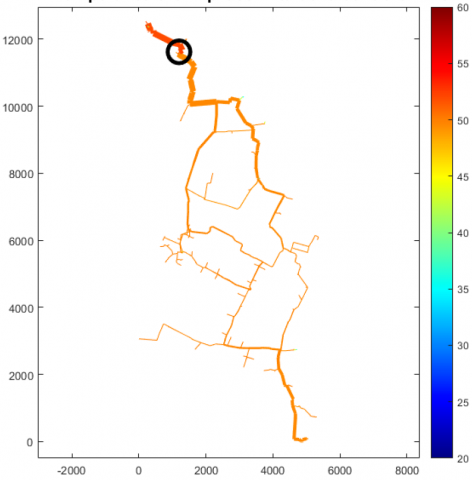Figure 10. Temperature distribution on the return network when a 12 MW heat pump is installed close to the Torino Nord plant

Figures 10 and 11 show two different installations of a 12 MW heat pump on the return network of the Turin district heating. A spring day is considered in the simulation, therefore the undisturbed (without heat pump) temperature is about 50 °C. In order to evaluate the optimal installation, various effects should be considered to perform proper evaluations:

(1) The heat pump pre-heats water, which thus reaches the plant at a higher temperature. This temperature affects the heat transfer in the cogeneration plants, which becomes less effective as the temperature increases. An installation far from the plant should be thus preferred from this point of view.

(2) A higher temperature involves larger thermal losses on the network, which make an installation close to the plants preferable.

(3) When the heat pump is installed in large pipes, where a large mass flow rate flows, the temperature variation is smaller, therefore the COP of the heat pumps is larger.

(4) The effect of a heat pump in terms of primary energy savings, depends on the primary energy factor of the plant which is substituted. In the case the heat pump allows reducing the operation of heat-only boilers, the impact is higher than in the case of cogeneration systems.

(5) As thermal load changes during the day and along the heating season, the use of a portion of pipeline might significantly change. As an example, a pipeline directed to a plant equipped with heat-only boiler is rarely used (as shown in figure 1). In this case, the use of a heat pump would be limited being constrained by the use of the plant.

In the scenarios shown in figures 8 and 9, the thermal plant located in the north side of the network is supplying the largest portion of thermal load. The heat pump is here used in order to allow the cogeneration plant reducing the thermal generation and increase the electricity production. Water temperature reaching the plant is about 48 °C in the first case and 47.8 °C in the second case. This means that the impact on the heat exchange effectiveness is lower in the second case. Concerning thermal losses on the return network, these are about 4.27 MW and 4.35 MW respectively. These impacts are quite small due to the limited size of the heat pump, which covers in this case less than 2% of the nominal thermal load; when larger power-to-heat systems are considered, deviations become significant.

Similar effects can be observed in other applications, such as the installation/operation of thermal storage units or when operating strategies involving actions on multiple plants or pumping systems should be examined. In these cases, the proposed approach can be used as a reliable tool for the optimization of the different options thanks to the significant reduction in computational time (which increases with the network size) and the reduced deviations in the results.

## 11.png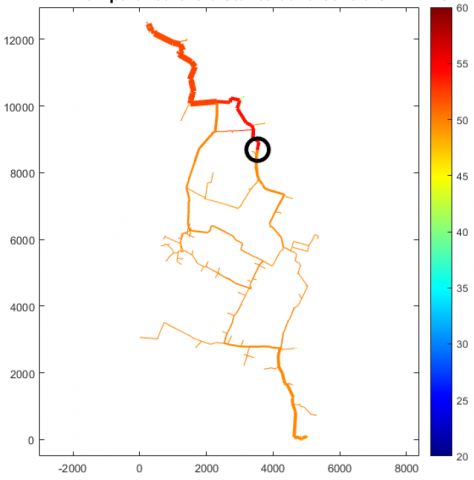Figure 11. Temperature distribution on the return network when a 12 MW heat pump is installed in the vicinity of Politecnico plant

7. Conclusions

In this paper, a modeling approach for fast simulation of large district heating networks is proposed. The model is composed by two reductions techniques which are combined together: 1) fluid flow model reduction and 2) thermal model reduction. The technique applied to the fluid flow model consists in transforming loop networks into three-shape networks. This is particularly useful for transport networks, where loops are frequent. The main idea consists in removing to a loop branch and substituting proper boundary conditions in the inlet and outlet nodes of this branch. These conditions are obtained using a black box model which is trained using full simulations corresponding to different operating conditions.

The technique applied to the thermal model is suitable for the application to distribution networks. As a result, the full network is represented as composed by the full model of the transport network and a reduced model of the various distribution networks. Reduction is obtained by applying a clustering technique to the buildings connected to each distribution network, so that the network is treated as a limited number of parallel branches, each connecting a cluster of buildings to the transport network.

The approach is tested considering the Turin district heating network. Results show that the average deviations on the mass flow rates are lower than 0.3%, while the deviations on the temperatures are lower than 4%. The latter can be further reduced by optimizing the clustering approach. In both cases reductions in computational time are of three orders of magnitude. The proposed approach is thus shown to represent and effective approach for DH optimizations both at a design and operation level able to take the effects of network topology into account.

Acknowledgments

This work has been conducted within the H2020 Project PLANET: Planning and operational tools for optimising energy flows & synergies between energy networks. Grant agreement 773839. https://www.h2020-planet.eu/

Authors thanks employers of IREN company for the support and data.

References

 Lund H, Østergaard PA, Chang M, Werner S, Svendsen S, Sorknæs P, Thorsen JE, Hvelplund F, Gram Mortensen BO, Vad Mathiesen B, Bojesen C, Duic N, Zhang X, Moller B. (2018). The status of 4th generation district heating: Research and results. Energy 164: 147-159. https://doi.org/10.1016/j.energy.2018.08.206

 Buffa S, Cozzini M, D’Antoni M, Baratieri M, Fedrizzi R. (2019). 5th generation district heating and cooling systems: A review of existing cases in Europe. Renewable and Sustainable Energy Reviews 104: 504-522. https://doi.org/10.1016/j.rser.2018.12.059

 Schmidt D, Kallert A, Blesl M, Svendsen S, Li HW, Nord N, Sipilä K. (2018). Low temperature district heating for future energy systems. Energy Procedia 116: 9-12. https://doi.org/10.1016/j.egypro.2017.05.052

 Lund H, Werner S, Wiltshire R, Svendsen S, Thorsen JE, Hvelplund F, Vad Mathiesen B. (2014). 4th Generation District Heating (4GDH) Integrating smart thermal grids into future sustainable energy systems. Energy 68: 1-11. https://doi.org/10.1016/j.energy.2014.02.089

 Ziemele J, Kalnins R, Vigants G, Vigants E, Veidenbergs I. (2018). Evaluation of the industrial waste heat potential for its recovery and integration into a fourth generation district heating system. Energy Procedia 147: 315-321. https://doi.org/10.1016/j.egypro.2018.07.098

 Mancarella P. (2014). MES (multi-energy systems): An overview of concepts and evaluation models. Energy 65: 1-17. https://doi.org/10.1016/j.energy.2013.10.041

 Hennessy J, Li H, Wallin F, Thorin E. (2018). Towards smart thermal grids: Techno-economic feasibility of commercial heat-to-power technologies for district heating. Applied Energy 228: 766-776. https://doi.org/10.1016/j.apenergy.2018.06.105

 Benonysson A, Bøhm B, Ravn HF. (1995). Operational optimization in a district heating system. Energy Conversion and Management 36(5): 297-314. https://doi.org/10.1016/0196-8904(95)98895-T

 Brundu FG, Patti E, Osello A, Del Giudice M, Rapetti N, Krylovskiy A, Acquaviva A. (2017). IoT software infrastructure for energy management and simulation in smart cities. IEEE Transactions on Industrial Informatics 13(2): 832-840. https://doi.org/10.1109/TII.2016.2627479

 Guelpa E, Barbero G, Sciacovelli A, Verda V. (2017). Peak-shaving in district heating systems through optimal management of the thermal request of buildings. Energy 137: 706-714. https://doi.org/10.1016/j.energy.2017.06.107

 Verda V, Guelpa E, Sciacovelli A, Acquaviva A, Patti E. (2016). Thermal peak load shaving through users request variations. International Journal of Thermodynamics 19(3): 168-176.

 Wang H, Yin W, Abdollahi E, Lahdelma R, Jiao W. (2015). Modelling and optimization of CHP based district heating system with renewable energy production and energy storage. Applied Energy 159: 401-421. https://doi.org/10.1016/j.apenergy.2015.09.020

 da Cunha JP, Eames P. (2016). Thermal energy storage for low and medium temperature applications using phase change materials-a review. Applied Energy 177: 227-238. https://doi.org/10.1016/j.apenergy.2016.05.097

 Keçebaş A, Yabanova İ. (2012). Thermal monitoring and optimization of geothermal district heating systems using artificial neural network: A case study. Energy and Buildings 50: 339-346. https://doi.org/10.1016/j.enbuild.2012.04.002

 Guelpa E, Toro C, Sciacovelli A, Melli R, Sciubba E, Verda V. (2016). Optimal operation of large district heating networks through fast fluid-dynamic simulation. Energy 102: 586-595. https://doi.org/10.1016/j.energy.2016.02.058

 Harary F. (1995). GraphTheory.Narosa Publishing House, New Delhi.

 Patankar S. (1980). Numerical Heat Transfer and Fluid Flow. CRC Press.

 Guelpa E, Toro C, Sciacovelli A, Melli R, Sciubba E, Verda V. (2016). Optimal operation of large district heating networks through fast fluid-dynamic simulation. Energy 102: 586-595. https://doi.org/10.1016/j.energy.2016.02.058